• 基于载波抑制双边带调制的微波光子移相器，侯雪缘，石宇笛，本文提出了一种基于载波抑制双边带调制的微波光子移相器。双平行马赫-曾德尔调制器（DPMZM）上臂输出光载波信号，DPMZM下臂加载射频�
• 此matlab代码，给出了现代通信原理中的常规双边带调幅(AM)的matlab仿真程序，给出了调制与解调的过程，并分析了在不同信噪比条件下的解调性能。
• 　所谓双边带调制（DSB）即载波抑制的调幅调制，它的载波被抑制，包络与调制前的信号不同，利用这一点即可实现信号的加密。加密时，将发话方语音信号调制在一个单音频信号上，从而产生一个经过DSB调制的音频信号（该...
• DBL 调制：它是一种线性调制，包括根据信息或调制信号的变化来修改载波信号的幅度。 双边带调制等效于AM调制，但没有载波重新插入。matlab
• 在中心站采用强度调制将射频信号调制到光载波上产生一个双边带信号,滤掉中心载波后,利用光交叉复用器把双边带信号的上下边模分开,将数据速率为2.5 Gbit/s的基带信号调制到下边模后,再与未调信号耦合后产生光毫米...
• 幅度调制(线性调制)是由调制信号去控制高频载波的幅度，使之调制信号的频谱线性变化。载波信号：$c(t) = A\cos\omega_ct$，基带信号为m(t)，则已调信号为：(设基带信号m(t)的频谱为$M(\omega)$)$$s_m(t)=Am(t)\cos... 幅度调制(线性调制)是由调制信号去控制高频载波的幅度，使之调制信号的频谱线性变化。 载波信号： c(t) = A\cos\omega_ct ，基带信号为m(t)，则已调信号为：(设基带信号m(t)的频谱为M(\omega))$$ s_m(t)=Am(t)\cos\omega_ct  S_m(\omega)= \frac{A}{2}[M(\omega + \omega_c) + M(\omega - \omega_c) ] $$可以看到，幅度调制就是把基带信号的频谱搬移到\omega_c处，再乘以1/2 。是线性变换。 AM调制$$s_{AM}(t) =[A_0+m(t)]\cos\omega_ct S_{AM}=\pi A_0 [ \delta(\omega + \omega_c) + \delta(\omega - \omega_c) ] + \frac{1}{2}[M(\omega + \omega_c) + M(\omega - \omega_c) ] 

为使用包络检波的方式进行解调，要求 $|m(t)|<=A_0$

clear all;

%% AM调制

fs = 800; % 采样速率，单位kHz

dt=1/fs; % 采样时间间隔，单位ms

T = 200; % 采样的总时间。频谱分辨率(df=1/T)。

t = 0 : dt : T-dt;

fm = 1; % 调制信号的频率，单位kHz

fc = 10; % 载波信号的频率，单位kHz

m = cos(2*pi*fm*t); % 调制信号

A = 3; %直流信号

s = (m+A).*cos(2*pi*fc*t); %已调信号

[f,sf] = T2F(t,s);

figure(1)

plot(t,s);

axis([0,2,-4,4]);

figure(2)

plot(f,abs(sf));

axis([-15,15,0,max(abs(sf))]);

DSB调制$s_{DSB}(t) =m(t)\cos\omega_ct$  $S_{DSB}(\omega)=\frac{1}{2}[M(\omega + \omega_c) + M(\omega - \omega_c) ]$，只能用相干解调

clear all;

%% DSB调制

% DSB(双边带)只需将调制信号m(t)与载波信号cos(wt)直接相乘即可

dt=1/800;

T = 200; % 采样的总时间。频谱分辨率(df=1/T)。

t = 0 : dt : T-dt;

fm = 2; % 调制信号的频率，单位kHz

fc = 20; % 载波信号的频率，单位kHz

m = cos(2*pi*fm*t); % 调制信号

s = m.*cos(2*pi*fc*t); %DSB已调信号

[f,sf] = T2F(t,s);

figure(1)

plot(t,s);

axis([0,1,-1,1]);

figure(2)

plot(f,abs(sf));

axis([-30,30,0,55]);

其中的函数T2F是信号的傅里叶变换

%% 函数 计算信号的傅里叶变换

function[f, sf] = T2F(t,st) % t为时域采样点；st为采样的时域信号

dt = t(2) -t(1);

% T = t(end);

T = t(end)-t(1)+dt;

df = 1/T;

N = length(st);

f = -N/2*df : df: N/2*df - df;

sf = fft(st);

sf = T/N * fftshift(sf);

end

还有F2T傅里叶反变换

%% 计算信号频谱 sf 的傅里叶反变换

function [ t, st ] = F2T( f, sf )

df = f(2)-f(1);

Fmx = f(end)-f(1)+df;

dt = 1/Fmx;

N = length(sf);

T = dt * N;

t = 0 : dt : T-dt; % 或 t = -T/2 : dt : T/2-dt;

sff = fftshift(sf);

st = Fmx * ifft(sff);

end

展开全文• 当信号间存在频差时双边带信号互相关对测距影响的研究较少。首先从信号接收的角度，给出双边带信号复用技术的简化模型。在此基础上，分析不同频信号互相关对伪码和载波跟踪的影响，最后通过仿真数据验证了结论的正确...
• 双边带幅度调制及其MATLAB仿真科 技 交 流张洁 王赋攀1 2四川农业大学 工程技术学院1.计算机科学与技术系 雅安625014西南科技大学 计算机科学与技术学院双边带幅度调制及其MATLAB仿真 2.绵阳 )621010调制解调技术...

双边带幅度调制及其MATLAB仿真

科 技 交 流

张洁 王赋攀

1 2

四川农业大学 工程技术学院

1.

计算机科学与技术系 雅安625014

西南科技大学 计算机科学与技术学院

双边带幅度调制及其MATLAB仿真 2.

绵阳 )

621010

调制解调技术直接决定着通信系统质量的好坏,是通信系统中的一个重要研究方向。双边带幅

[摘 要]

在计算机上实现对双边带幅度调制过程仿真,从而为实际应用和设计通信系统提供了一个便捷、直观的平台。

通信 双边带幅度调制

现代通信系统要求通信距离远、通信容量大、传输质量好。作 图 典型的幅度调制波形

1

为其关键技术之一的调制解调技术一直是人们研究的一个重要方向。 2、双边带幅度调制及其MATLAB仿真

从模拟调制到数字调制,从二进制调制发展到多进制调制,虽然调 双边带幅度调制 幅度调制往往是线性调制。常用的线性调

2.1 :

制方式多种多样,但都是朝着使通信系统更高速、更可靠的方向发 制方法包括双边带幅度调制 ( )、常规幅度调制和单边幅度

DSB-AM

展。一个系统的通信质量,很大程度上依赖于所采用的调制方式。 调制 ( )。双边带幅度调制 ( ),又称抑制载波调

SSB-AM DSB-AM

因此对调制方式的研究直接决定着通信系统质量的好坏。 幅,其特点是已调制信号频谱中包含两个边带 (上、下边带),且这

实际的通信系统需要完成从信源到信宿的全部功能,这通常是 两个边带包含相同的信息。在 DSB-AM中已调信号的时域表示如

比较复杂的。对这个系统做出的任何改动 (如改变系统的结构、改 下:

变某个参数的设置等)都可能影响整个系统的稳定性和性能。因此

在设计新系统、对原有的系统做出修改或者进行相关研究时,通常

要进行建模和仿真,通过仿真结果来衡量方案的可行性,从中选择 式中 是消息信号, 是载波, 是载波频率。

m (t)

展开全文• % (ExampleDSBdemfilt.m)% This program uses triangl.m to illustrate DSBmodulation% and demodulationts=1.e-4;t=-0.04:ts:0.04;Ta=0.01;m_sig=triangl((t+0.01)/0.01)-triangl((t-0.01)/0.01);...

% (ExampleDSBdemfilt.m)

% This program uses triangl.m to illustrate DSB

modulation

% and demodulation

ts=1.e-4;

t=-0.04:ts:0.04;

Ta=0.01;

m_sig=triangl((t+0.01)/0.01)-triangl((t-0.01)/0.01);

Lm_sig=length(m_sig);

Lfft=length(t); Lfft=2^ceil(log2(Lfft));

M_fre=fftshift(fft(m_sig,Lfft));

freqm=(-Lfft/2:Lfft/2-1)/(Lfft*ts);

B_m=150;  % Bandwidth of

the signal is B_m Hz.

h=fir1(40, [B_m*ts]);

t=-0.04:ts:0.04;

Ta=0.01;fc=300;

s_dsb=m_sig.*cos(2*pi*fc*t);

Lfft=length(t); Lfft=2^ceil(log2(Lfft)+1);

S_dsb=fftshift(fft(s_dsb,Lfft));

freqs=(-Lfft/2:Lfft/2-1)/(Lfft*ts);

%  Demodulation begins by multiplying with

the carrier

s_dem=s_dsb.*cos(2*pi*fc*t)*2;

S_dem=fftshift(fft(s_dem,Lfft));

% Using an ideal LPF with bandwidth 150 Hz.

s_rec=filter(h,1,s_dem);

S_rec=fftshift(fft(s_rec,Lfft));

Trange=[-0.025 0.025 -2 2];

figure(1)

subplot(221);td1=plot(t,m_sig);

axis(Trange); set(td1,'Linewidth',1.5);

xlabel('{\it t} (sec)'); ylabel('{\it m}({\it t})');

title('Message signal');

subplot(222);td2=plot(t,s_dsb);

axis(Trange); set(td2,'Linewidth',1.5);

xlabel('{\it t} (sec)'); ylabel('{\it s}_{\rm

DSB}({t})');

title('DSB-SC modulated signal');

subplot(223);td3=plot(t,s_dem);

axis(Trange); set(td3,'Linewidth',1.5);

xlabel('{\it t} (sec)'); ylabel('{\it e}({\it t})');

title('{\it e}({\it t})');

subplot(224);td4=plot(t,s_rec);

axis(Trange); set(td4,'Linewidth',1.5);

xlabel('{\it t} (sec)'); ylabel('{\it m}_d({\it t})');

title('Recovered signal');

Frange=[-700 700 0 200];

figure(2)

subplot(221);fd1=plot(freqm,abs(M_fre));

axis(Frange); set(fd1,'Linewidth',1.5);

xlabel('{\it f} (Hz)'); ylabel('{\it M}({\it f})');

title('Message spectrum');

subplot(222);fd2=plot(freqs,abs(S_dsb));

axis(Frange); set(fd2,'Linewidth',1.5);

xlabel('{\it f} (Hz)'); ylabel('{\it s}_{\rm

DSB}({f})');

title('DSB-SC spectrum');

subplot(223);fd3=plot(freqs,abs(S_dem));

axis(Frange); set(fd3,'Linewidth',1.5);

xlabel('{\it f} (Hz)'); ylabel('{\it E}({\it f})');

title('Spectrum of {\it e}({\it t})');

subplot(224);fd4=plot(freqs,abs(S_rec));

axis(Frange); set(fd4,'Linewidth',1.5);

xlabel('{\it f} (Hz)'); ylabel('{\it M}_d({\it f})');

title('Recovered spectrum');

展开全文• 利用 CCS 和DSP 编程实现双边带调制（查表法） CCS介绍： CCS的全称是Code Composer Studio，它是美国德州仪器公司（Texas Instrument,TI）出品的代码开发和调试套件。TI公司的产品线中有一大块业务是数字信号...

CCS介绍：

CCS的全称是Code Composer Studio，它是美国德州仪器公司（Texas Instrument,TI）出品的代码开发和调试套件。TI公司的产品线中有一大块业务是数字信号处理器（DSP）和微处理器（MCU），CCS便是供用户开发和调试DSP和MCU程序的集成开发软件。

问题描述：

在ccs下实现一个基本的调幅系统，即将输入的两路数据, 一路是载波, 另外一路是基带
信号, 进行双边带调制, 然后再输出。

电脑环境：

1、Windows XP SP3（Windows10 下 VMware12 Pro中的虚拟机）
2、Code Composer Studio2.0 点这里下载，密码：fyij

步骤：

1、首先我们用matlab生成所需要的载波和基带信号的正弦系数表，请参见博客matlab生成正弦系数表，修改这两个文件的头部, 使之成为符合CCS 文件I/O 的要求格式。
载波信号的正弦系数表：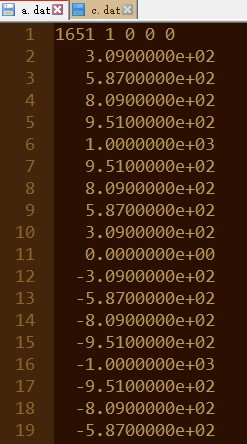基带信号的正弦系数表：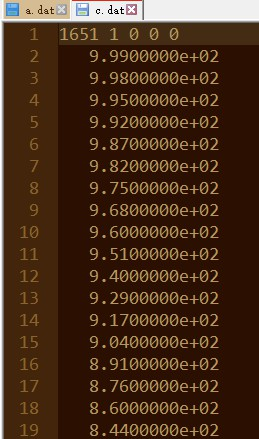2、首先将CCS设置为软件仿真模式（simulate），步骤如下gif动图所示。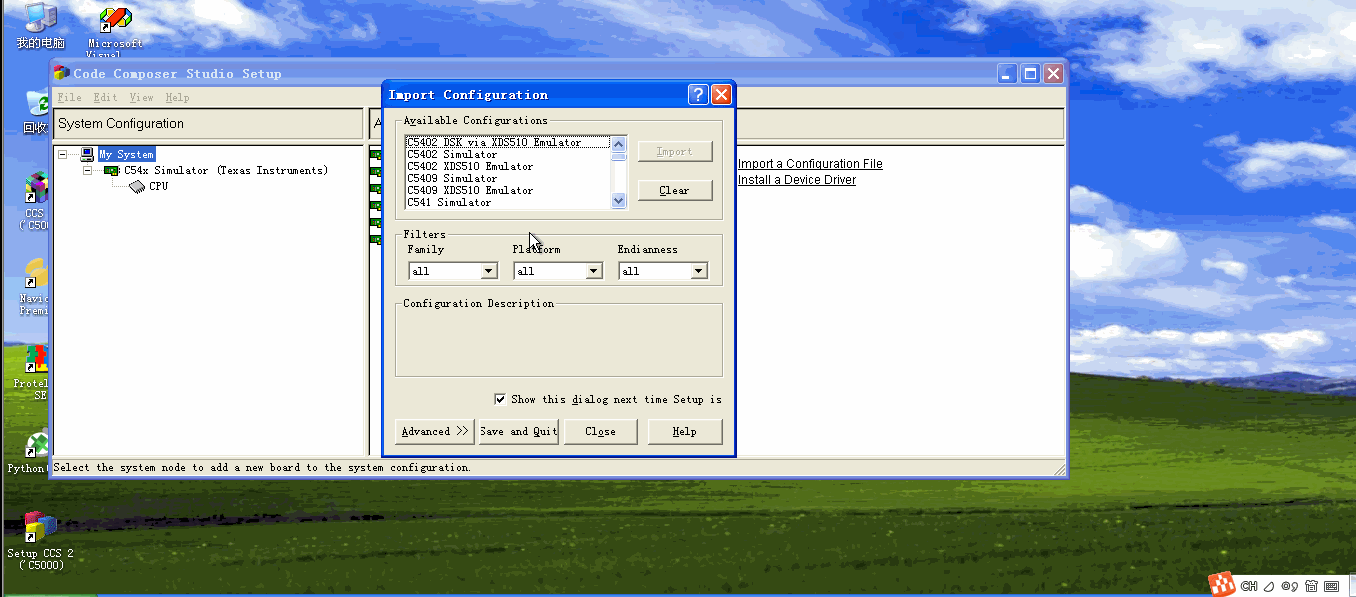3、打开ccs新建工程，并向工程中加入asm.cmd文件和ASMtest6.asm，步骤如下gif动图所示。CMD文件的作用请见博客CCS中CMD文件解析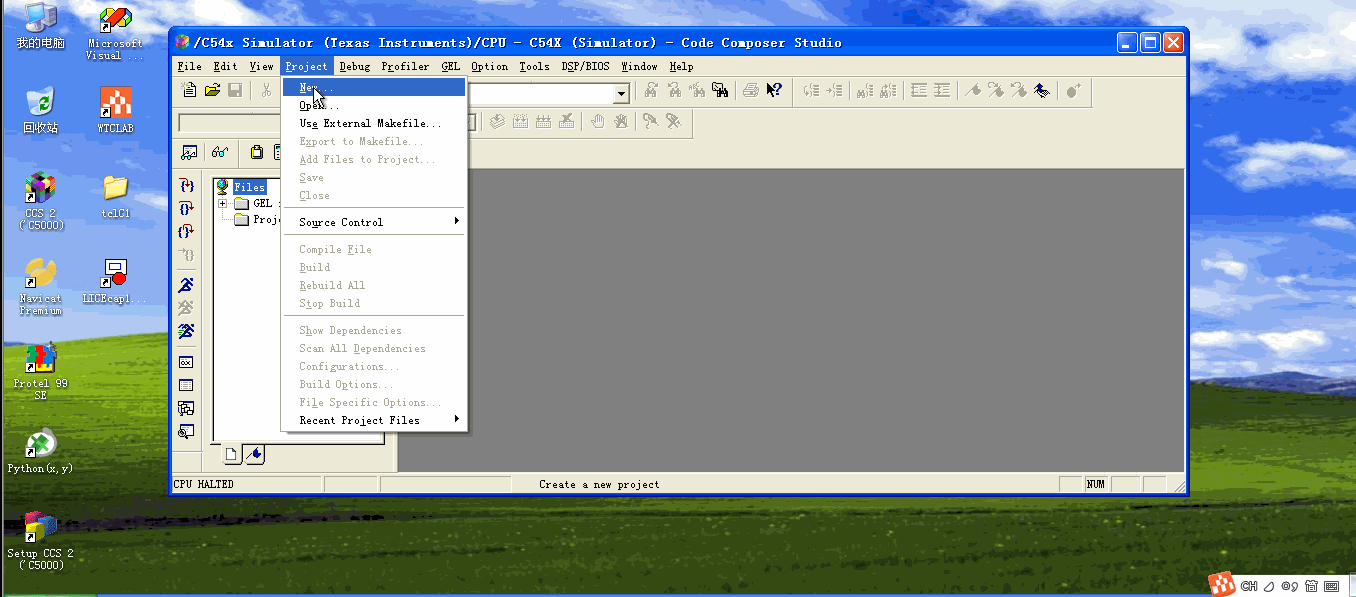asm.cmd文件代码如下：

MEMORY
{
PAGE 0 :
STACK  :  origin = 0x0080,  length = 0x0080  /* stack space */
XFER   :  origin = 0x0100,  length = 0x0300  /* reserve 0x300 words for comm kernel */
XFERHDR:  origin = 0x0400,  length = 0x003a  /* reserve 0x3a words for kernel header */
FIFO   :  origin = 0x043a,  length = 0x0046  /* reserve 0x46 words for fifo */
VECTORS:  origin = 0x0480,  length = 0x0080  /* interrupt vectors */
INT_PM_DRAM :  origin = 0x0500,  length = 0x1b00  /* on-chip ram 5402 */
PAGE 1:
SCRATCH     :  origin = 00060h,  length = 00020h  /* page-0 scratch-pad */
INT_DM_RAM  :  origin = 0x2000,  length = 0x2000  /* on-chip ram 5402 */
}

SECTIONS
{
.text  : > INT_PM_DRAM PAGE 0

.stack : > INT_DM_RAM  PAGE 1
.bss   : > INT_DM_RAM  PAGE 1
.data  : > INT_DM_RAM  PAGE 1
}

ASMtest6.asm文件代码如下：

.mmregs         ;允许寄存器的符号表示
.def _c_int00   ;定义程序入口标号
carrier .set    1   ;载波输入端口
signal  .set    2   ;基带信号输入端口
DSBout  .set    3   ;DSB调制输出端口

.data       ;数据段 根据cmd文件,从2000H开始
BUFFER1 .word   0x0 ;(2000H)
BUFFER2 .word   0x0 ;(2001H)
BUFFER3 .word   0x0 ;(2002H)

.text           ;程序段开始
_c_int00:
STM #BUFFER1, AR2;
STM #BUFFER2, AR3;
STM #BUFFER3, AR4;

STM #299,BRC            ;BRC=299 块循环300次
;因为输入数据有300个样值
RPTB LOOP-1             ;块循环开始处
NOP                     ;在此加入探针,进行数据文件输入输出
NOP
PORTR   carrier,*AR2    ;读入载波数据
PORTR   signal,*AR3     ;读入基带数据
MPY *AR2,*AR3,A         ;处理数据,相乘
;乘积结果的最大值为1000×1000
;为6位16进制有符号数,其中2位进入了AH
;为了保证输出, 需将结果右移8bit,再保存
STL A, -8, *AR4         ;右移8bit并存放数据
PORTW   *AR4,DSBout     ;输出数据
;并再次读入下一个数据
LOOP:
NOP                 ;完成任务后程序进入空操作
B LOOP
.end

4、由于这里我们使用了 I/O 空间, 所以需要配置I/O 空间, 使得0x0000 到0x0003 地址是可读
写的，步骤如下gif动图所示。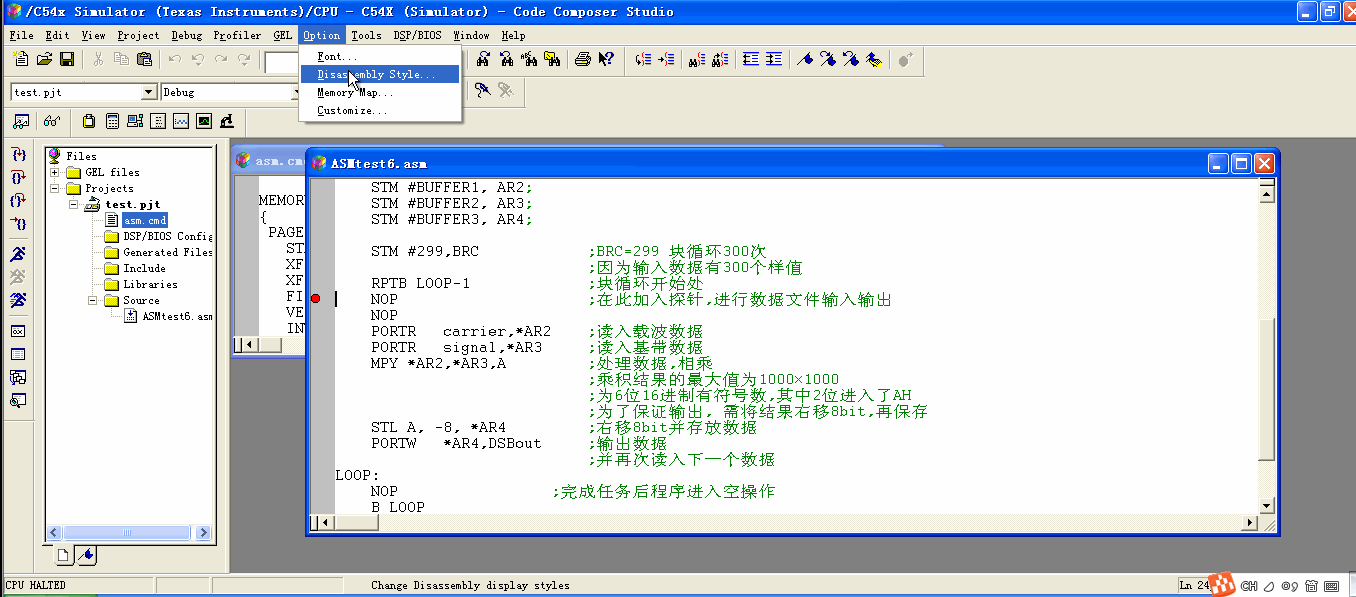5、然后将这 3 个I/O 空间地址与3 个文件相连接。即在 CCS 中编译，按F10单步运行程序。步骤如下gif动图所示。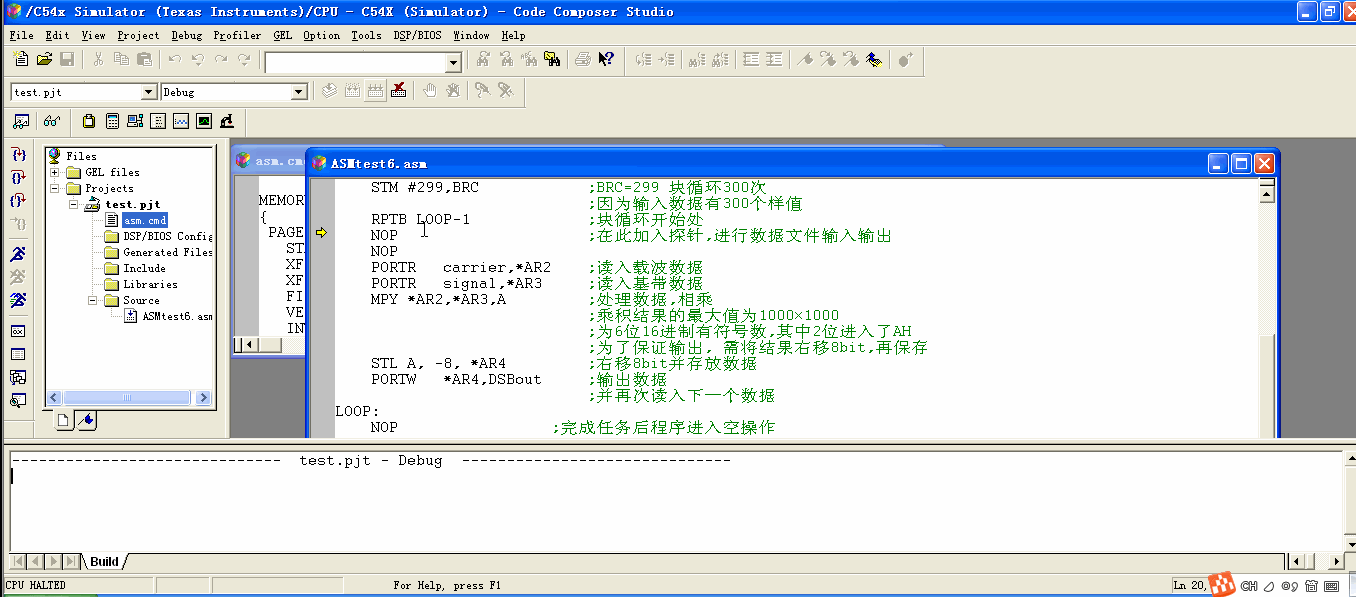6、观察程序无误之后，打开Data Memory 窗口和I/O Memory 窗口，以便观察。步骤如下gif动图所示。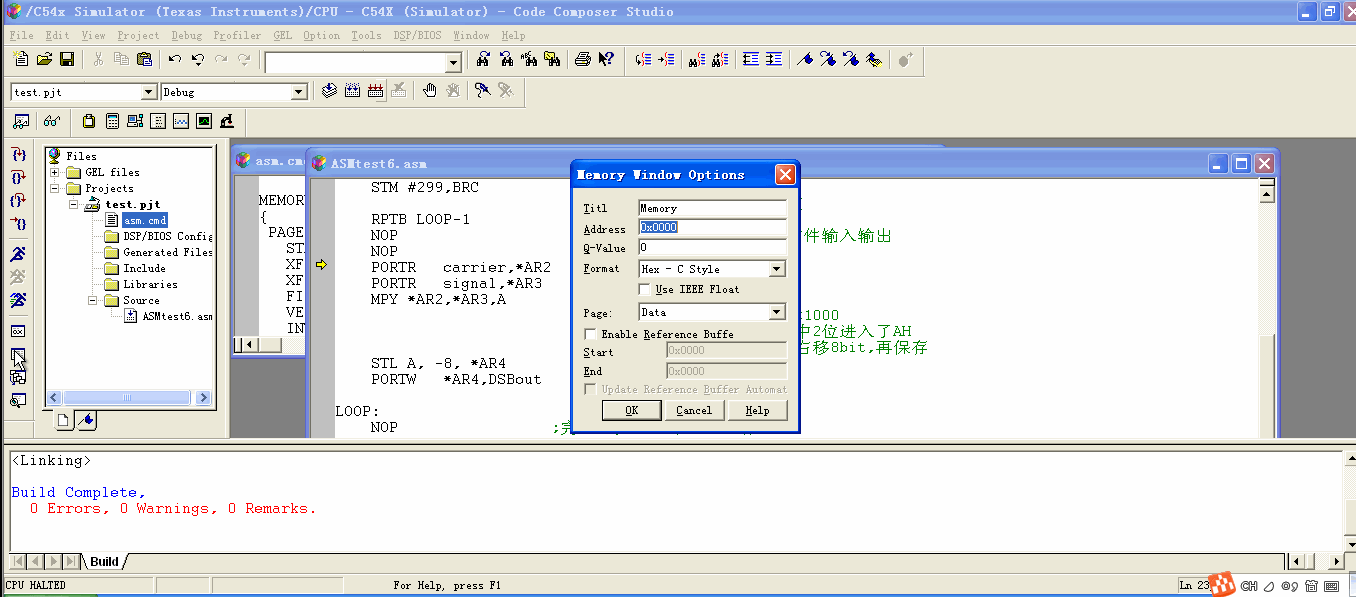7、在第21 行NOP 处加入断点和探针工具，进行数据文件输入输出，步骤如下gif动图所示。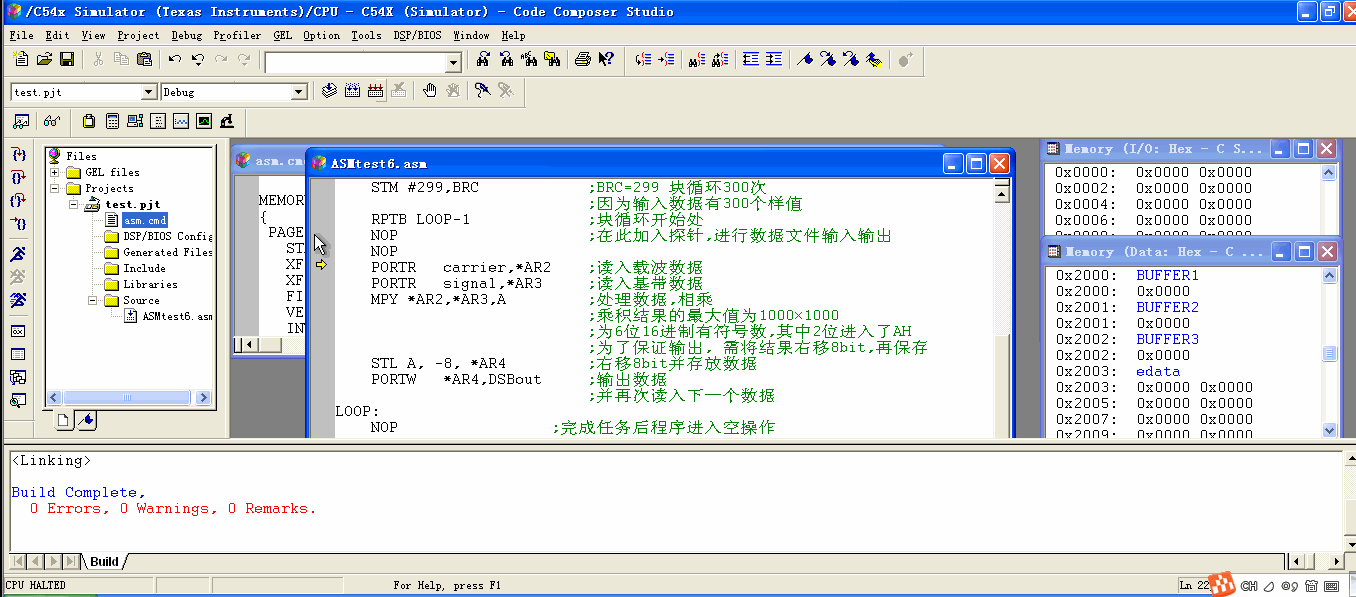8、在 CCS 的File->File I/O… 打开文件输入输出对话框，单击按钮 Add File 添加输入数据文件. 添加前面用matlab 产生并对文件头部作了修改的数据文件a.dat 和c.dat, 添加之后出现了2 个数据文件的控制对话框。如图，(Wrap Around 选项可不选，这样CCS 将数据文件中的数据读到结尾时就不再重新从头读起)。步骤如下gif动图所示。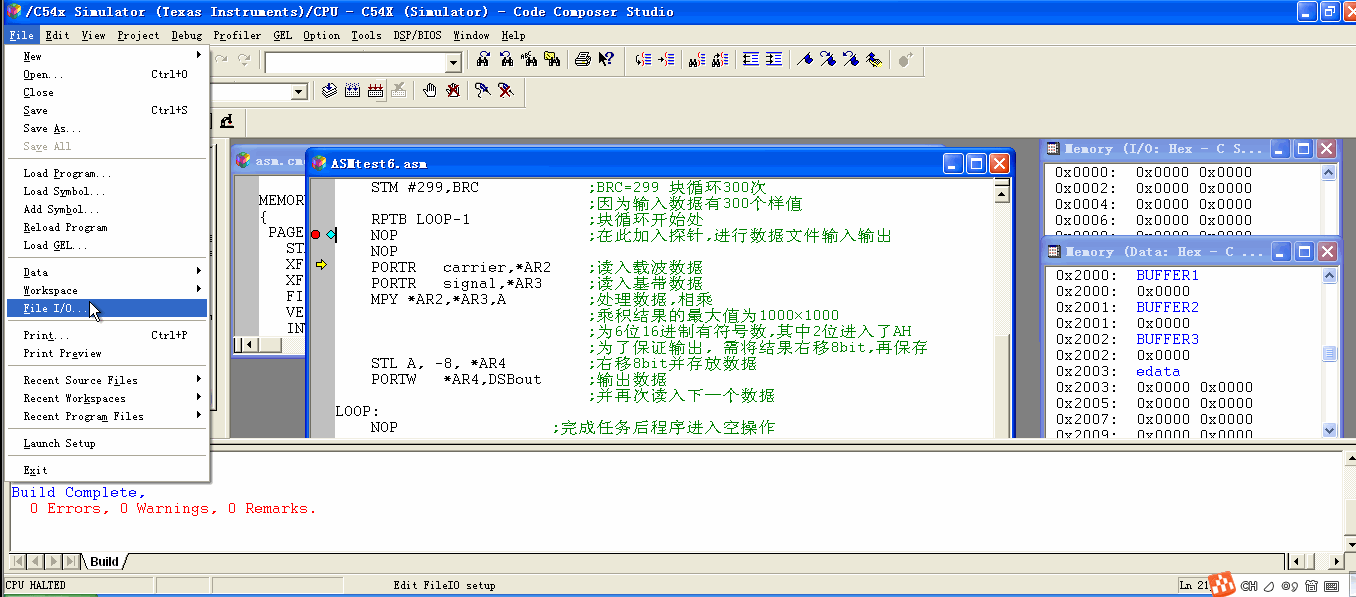文件格式选为 Interger 的dat 文件，在文件名栏中写入新文件名。步骤如下gif动图所示。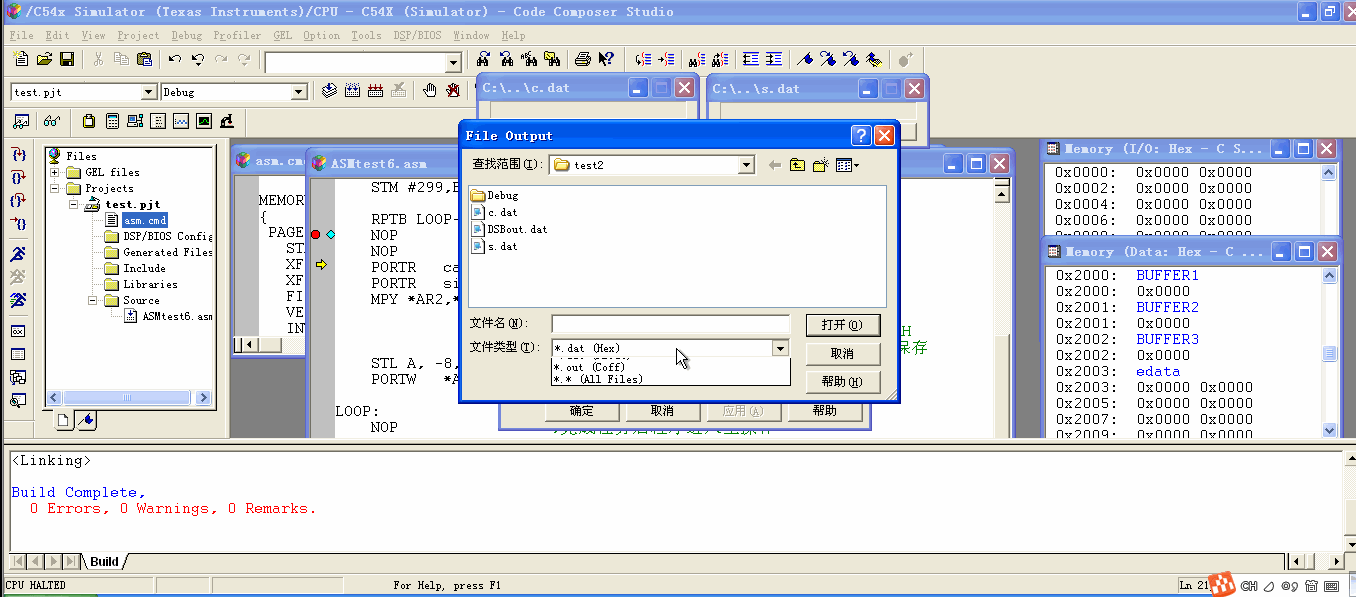注意, 第3 个数据文件输出控制对话框也出现了. 其中的显示“0”表示现在向该文件输出了0 个数据。

10、接下来的任务是将数据文件与探针点连接起来，即告诉CCS 在探针点处将数据从相
应文件中读入，并将相应数据输出给对应的数据文件。步骤如下gif动图所示。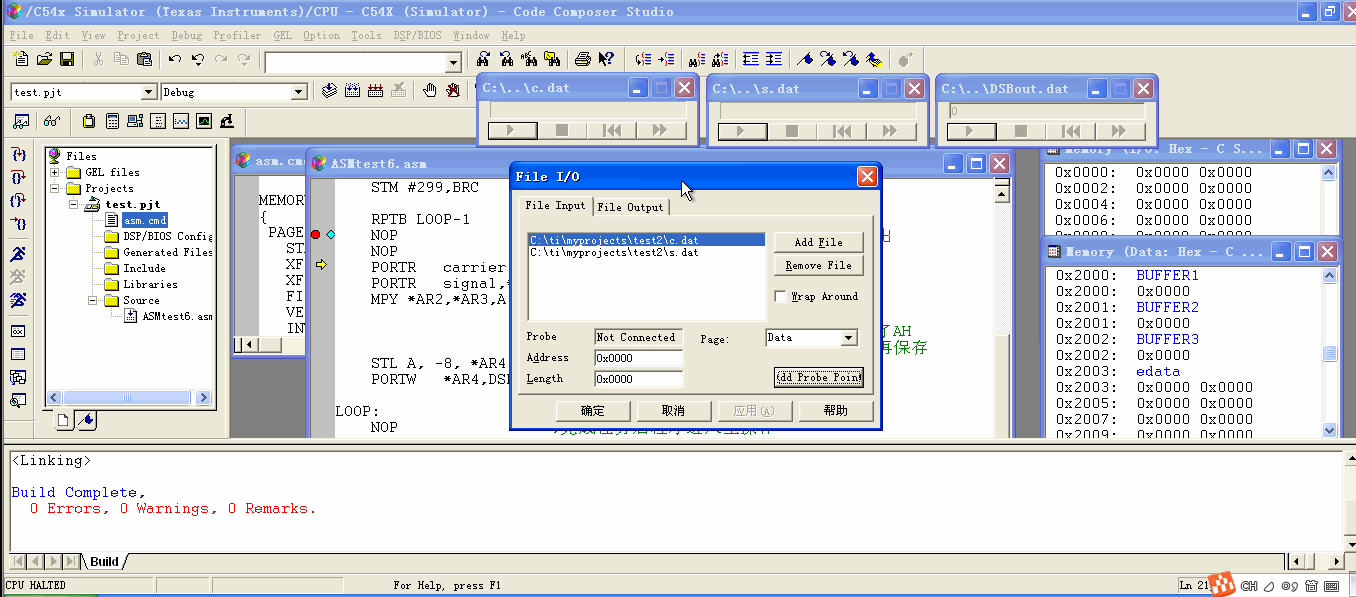11、完成后单击 OK 确定，这样就回到 CCS 主界面，单步执行程序到探针处，观察I/O 端口和数据内存中数据的变化，也可以动画方式执行程序，也可将断点去除， 按F5 执行程序完毕。
下图是执行程序到第33 次循环的结果。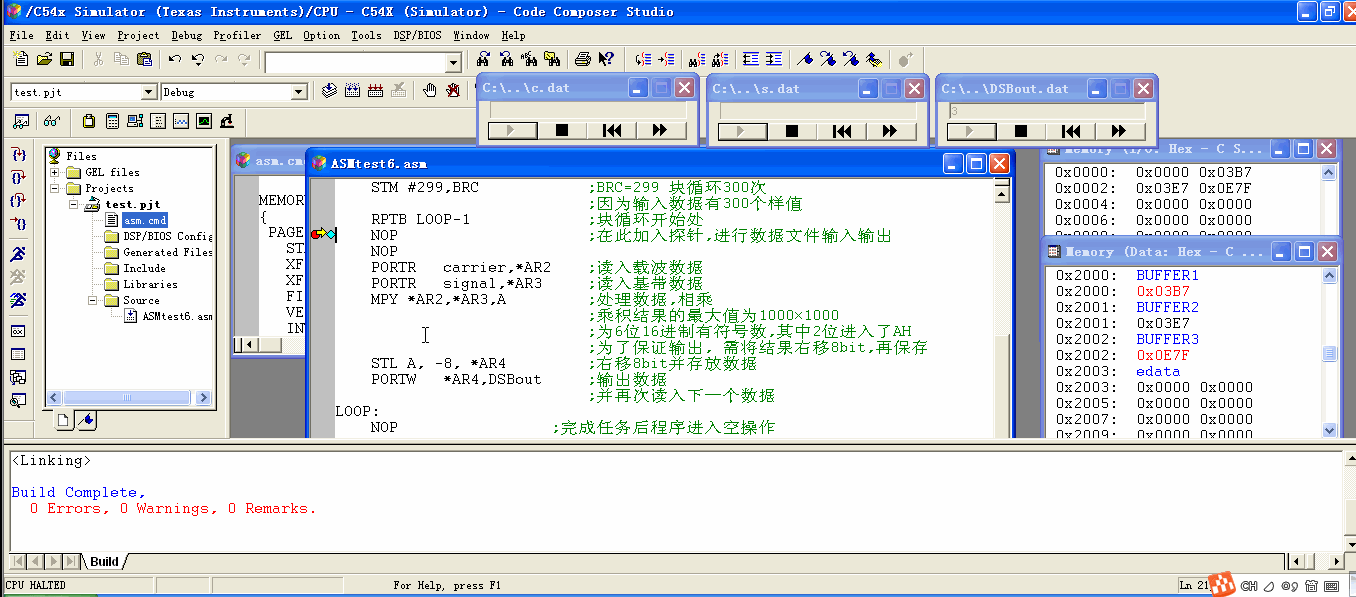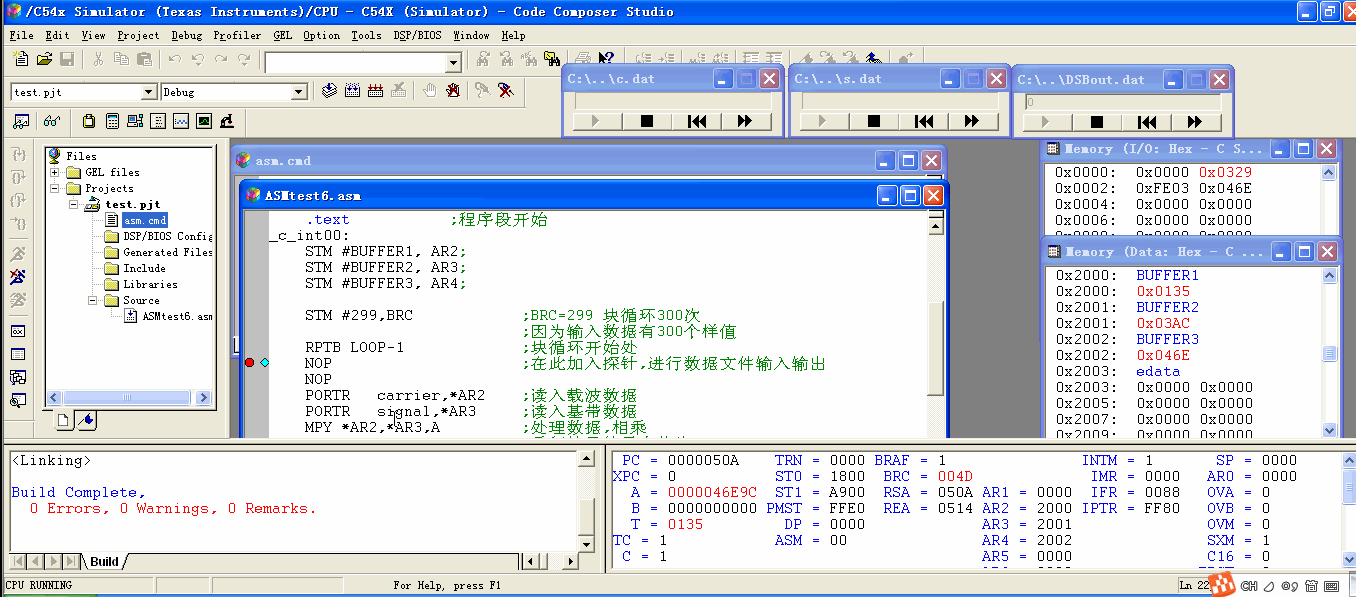12、利用CCS 的图示化功能直接看到输出波形。为了观察更长时间，我们可以将循环次数增
加，同时将File I/O 对话框中Input File 标签下两个输入文件的循环读入的选项“Wrap Around”选中即可。步骤如下gif动图所示。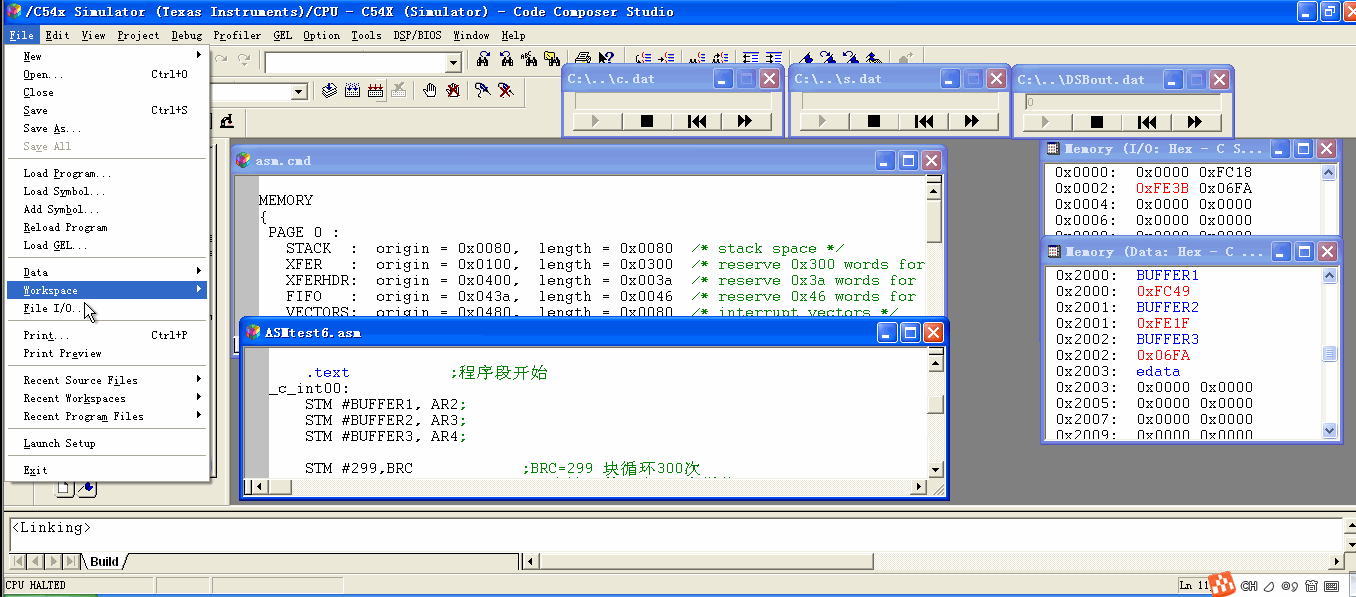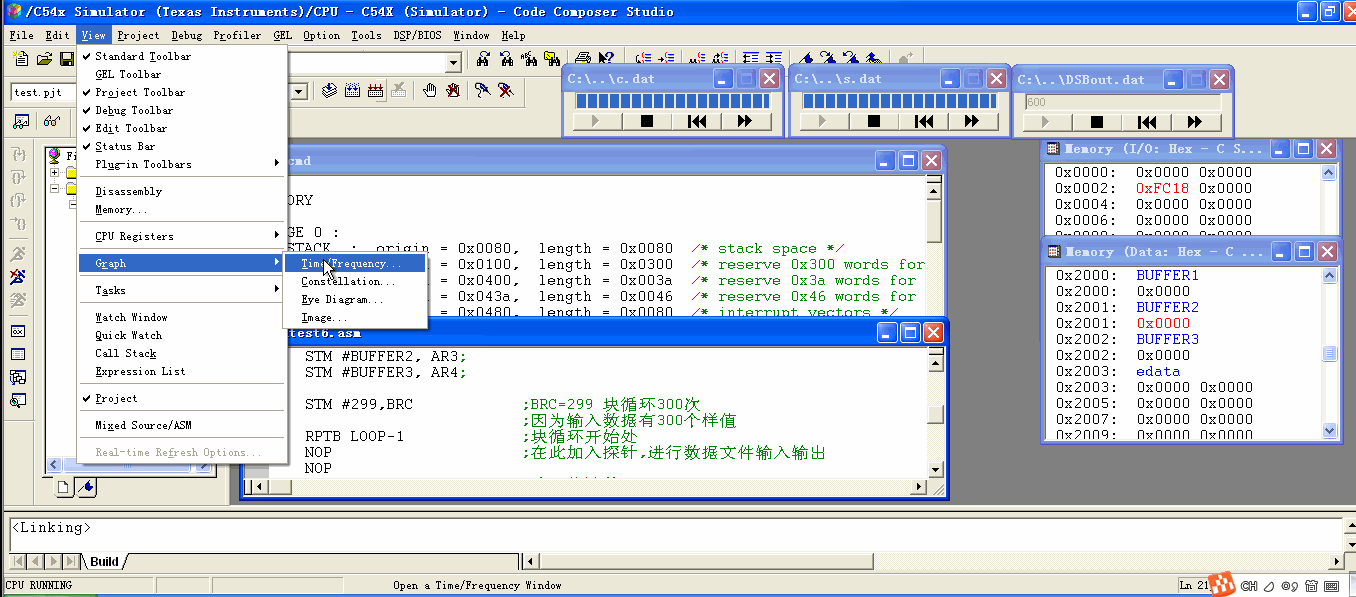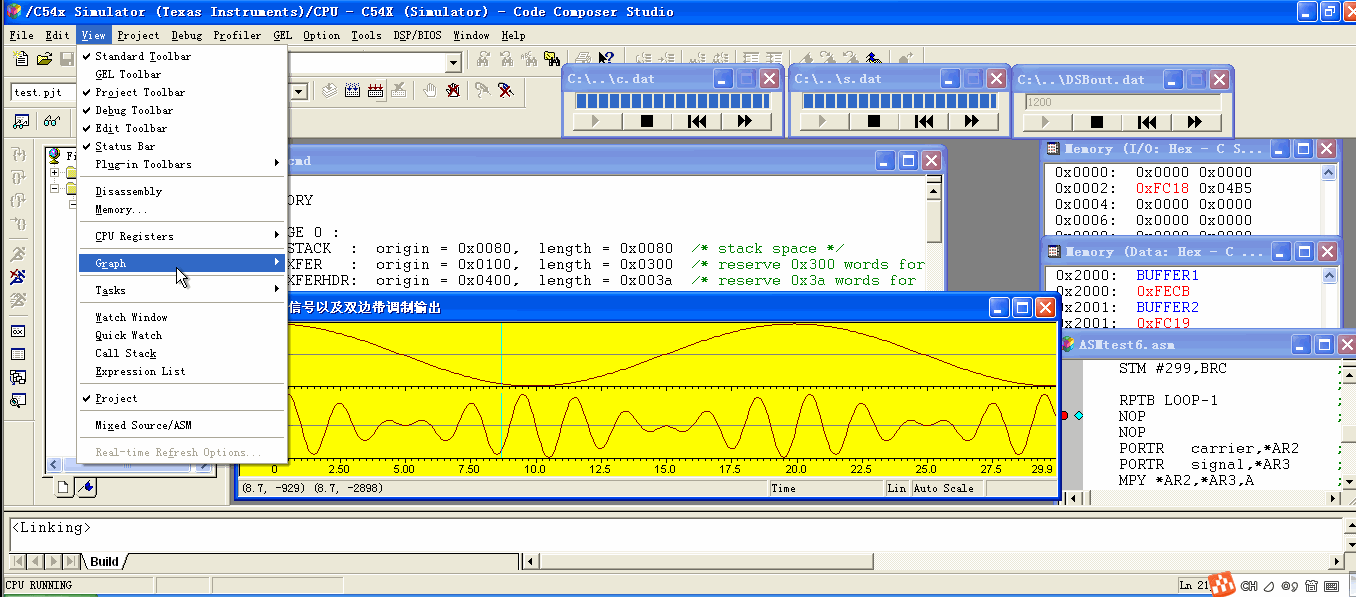13、利用CCS 的图示化功能直接看到功率谱。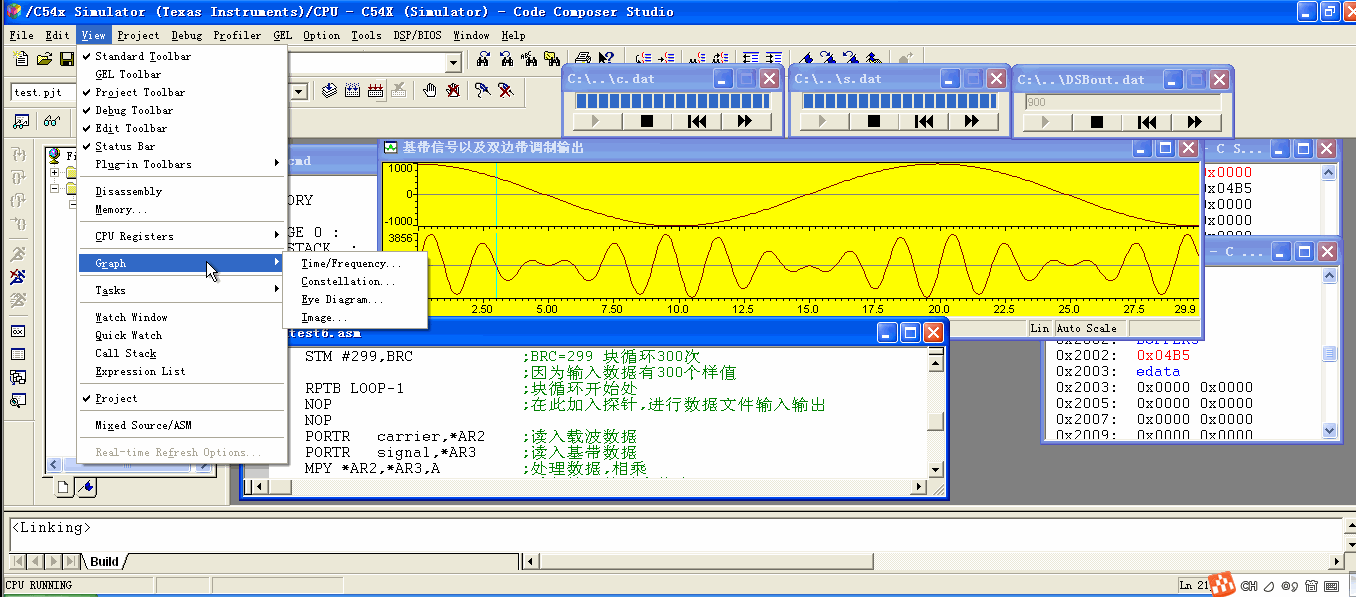总结

通过这次DSP作业，学习了如何使用ccs进行纯软件仿真。

展开全文ccs dsp 双边带调制 DSB调制
• DSB双边带信号调制，加噪声后解调，以及各个时刻的功率谱，频谱，自相关，时域波形。
• 幅度调制，载波频率=200 rad/sec 消息频率=3 rad/sec 载波和消息的幅度均为 1 调制信号 = A[1+ m(t)]cos(2*pi* Fc*t) 调制指数 = min[m(t)]/A 在本例中 =100%matlab
• 本帖最后由 安徽人1992 于 2016-7-16 16:11 编辑我在matlab上做一个双边带调幅和解调的例子，代码如下所示：>> a = 1;%基带信号为1Hz>> fc = 10;%载波信号为10Hz>> fs = 100;%采样频率为100Hz>...
• 武汉理工大学信号分析处理课程设计目录1基于MATLAB的抑制载波的双边带幅度调制(DSB)与解调分析摘要 .................................... 2 2、设计目的 ...........................................................
•研究论文
• 对一个模拟信号进行DSB调制分析，分析其频谱和瞬时幅度及瞬时相位
• Introduction In this lab, I know the principle of DSB/SSB modulation and demodulation and Hilbert transform. And I draw the LabVIEW program diagram for DSB/SSB modulation and demodulation....
• 1、系统描述 DSP 系统从I/O 端口将要处理的数据不断...（2）载波信号和基带信号及双边带调制输出参数设置： 载波信号和基带信号及双边带调制输出波形： （3）频谱参数设置： 频谱分析波形：DSB调制 CCS DSP
• 做通信实验时的必备指导书，包括实验一 信号源实验 实验二 信道与眼图实验 实验四 常规双边带调幅与解调实验（AM）实验七 频分复用实验（FDM）等几个通信必做实验，相信能够对您的学习有所助益！
• 文中介绍了一种双边PWM调制的数字D类放大器调制模块，使用伪自然采样法消除谐波失真。该伪采样算法是将牛顿-拉夫森迭代法和多项式逼近法相结合而形成的。近年来，虽有较多关于前沿PWM调制（LEPWM）和后沿PWM调制...
• 通过本地振荡器模拟双边带 (DSB) 调制和解调。 输入信号是您的声音，因此请对着麦克风讲话。 AWGN 噪声被添加到信号中。 过滤掉嘈杂的解调信号后，您可以听到接收到的信号。matlab
• 一种利用数字电路实现全载波双边带幅度调制信号的方法、电子技术,开发板制作交流AD9854 DDS FPGA
• 用MATLAB对DSB的调制解调进行仿真模拟，包括时域，频域，加噪，包络。 (MATLAB for DSB modulation and demodulation simulation, including time domain, frequency domain, noise, envelope.) 文件列表： DSB调制...
• 文章目录1、模拟调制基本原理2、 1、模拟调制基本原理 问题的提出   考虑我们用麦克风采集语音信号，完成声-电转换，得到的电信号频率范围在20Hz~20kHz之间，显然属于低通信号。语音（电）信号为模拟随机信号，......